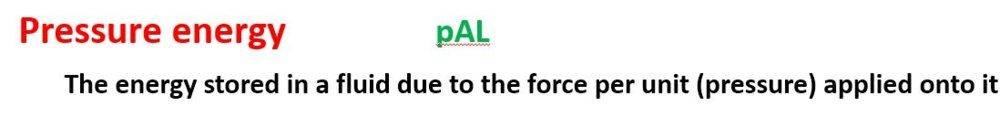# What is Pressure Energy & How to Calculate it?

tomtomtom1
Homework Statement:
What is Pressure Energy & How to Calculate it?
Relevant Equations:
What is Pressure Energy & How to Calculate it?
Hello all

I was hoping i could pick someones brain regarding Pressure Energy.

I was given the following piece of information that describes the Pressure Energy equation:-However i am not certain what each term means?

Would it be correct to state:-

Pressure Energy = Fluid Density * Area * Length
OR
Pressure Energy = Pressure * Area * Length
(The P could relate to pressure or density)

But i am not convinced that this is correct. The way i attempted to derive a pressure energy equation was by doing the following:- :-

Pressure = Force /Area

Since; Force * Distance = Work, i multiplied both upper and lower parts of the fraction by Distance

Pressure Energy = Force * Distance /Area * Distance

Pressure Energy = Work / Area * Distance

Since Area * Distance = Volume

I get:-

Pressure Energy = Work / Volume

But my pressure energy equation does not look like anything like the equation that was given to me.

Not sure what is going on?

Any ideas?

Thanks

Homework Helper
Gold Member
2022 Award
my pressure energy equation does not look like anything like the equation that was given to me.
Please post those two equations. (Maybe you have above, but it's not clear.)
Consider the pressure acting on an area. It generates a force. If that force were allowed to do work by advancing some distance normal to the area it would sweep out a volume. The "pressure energy" is the work per unit volume.
It is not, as I understand it, actual stored energy within a volume. Chet? @Chestermiller

Saptarshi Sarkar
Pressure = Force /Area

Since; Force * Distance = Work, i multiplied both upper and lower parts of the fraction by Distance

Pressure Energy = Force * Distance /Area * Distance

This is not correct. When you multiply by the same dimension both above and below, the dimension of the fraction does not change.

The dimension of pressure energy is the same as that of energy, i.e ML²/T². You should always try to use dimensional analysis to check your formulas as it is a very powerful tool in Physics.

Pressure = Force/Area = Force*Distance/Area*Distance = Pressure Energy/Volume

So, Pressure Energy = Pressure*Volume = pAL

Mentor
Please post those two equations. (Maybe you have above, but it's not clear.)
Consider the pressure acting on an area. It generates a force. If that force were allowed to do work by advancing some distance normal to the area it would sweep out a volume. The "pressure energy" is the work per unit volume.
It is not, as I understand it, actual stored energy within a volume. Chet? @Chestermiller
The term pressure energy has no particular physical meaning to me, although some people might refer to it as the term ##\frac{P}{\rho}## in the Bernoulli equation (to put it on a par with kinetic energy and potential energy).

•haruspex